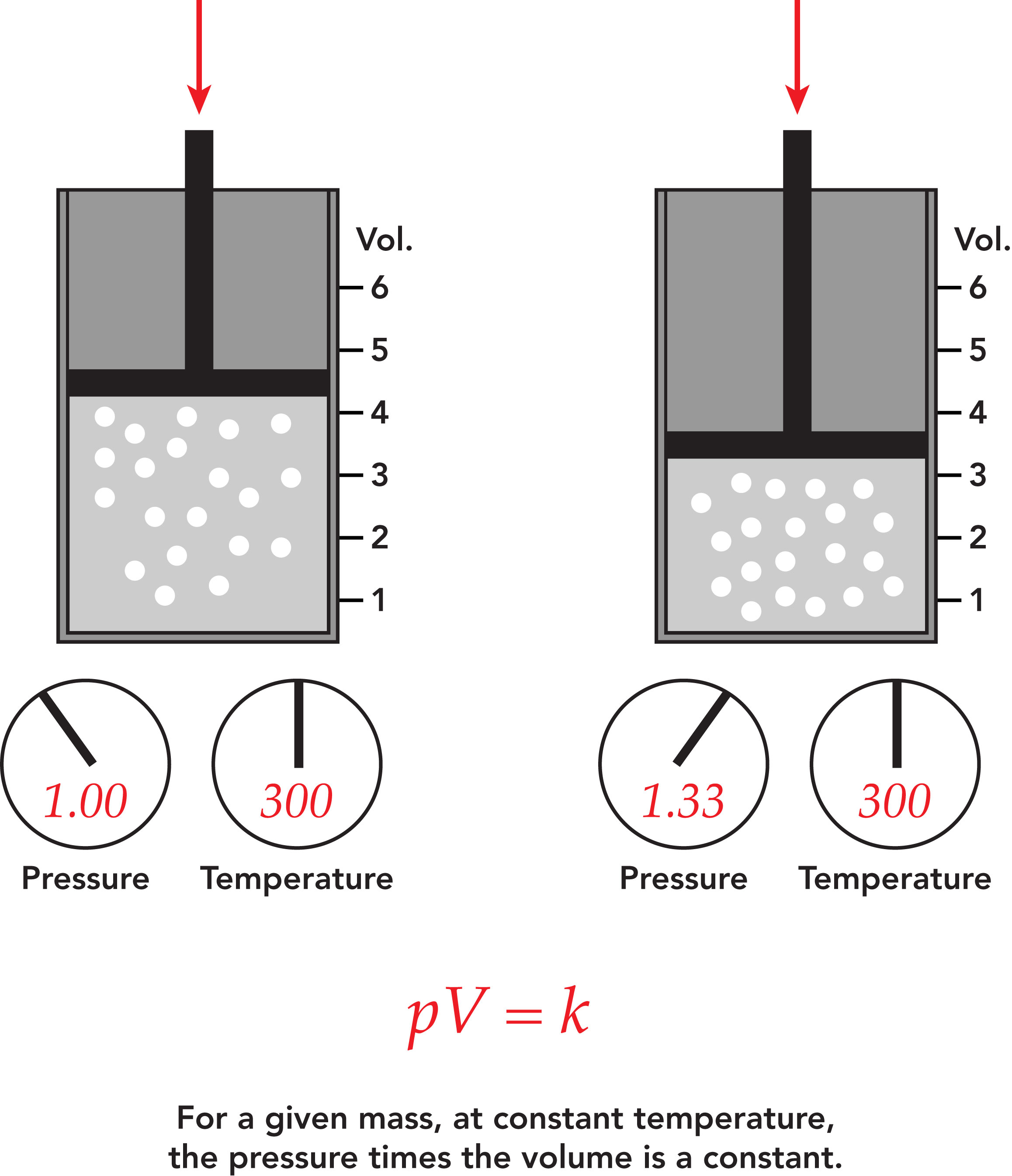Toll Free 877.245.6247

# Boyle's Law

Category:

Boyle’s Law is a basic law in chemistry describing the behavior of a gas held at a constant temperature. The law, discovered by Robert A. Boyle in 1662, states that at a fixed temperature, the volume of gas is inversely proportional to the pressure exerted by the gas. In other words, when a gas is pumped into an enclosed space, it will shrink to fit into that space, but the pressure that gas puts on the container will increase.

Perhaps a more straightforward way is to say Boyle's law is the relationship between pressure and volume. Mathematically, Boyle’s law can be written as pV=k, where p is the pressure of the gas, V is the volume of the gas, and k is a constant.

An example of Boyle’s law in action can be seen in a balloon. Air is blown into the balloon; the pressure of that air pushes on the rubber, making the balloon expand. If one end of the balloon is squeezed, making the volume smaller, the pressure inside increased, making the un-squeezed part of the balloon expand out. There is a limit to how much the air/gas can be compressed, however, because eventually the pressure becomes so great that it causes the balloon to break.